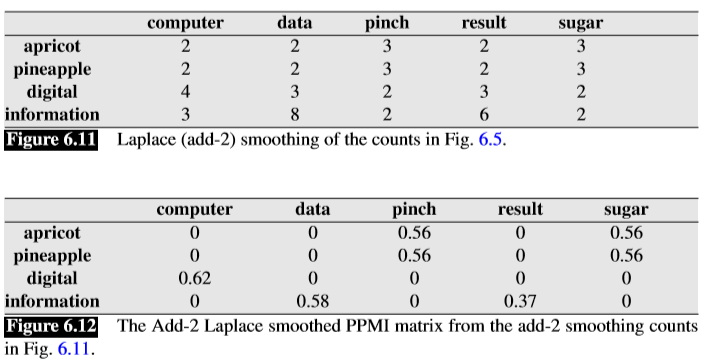### Mind Map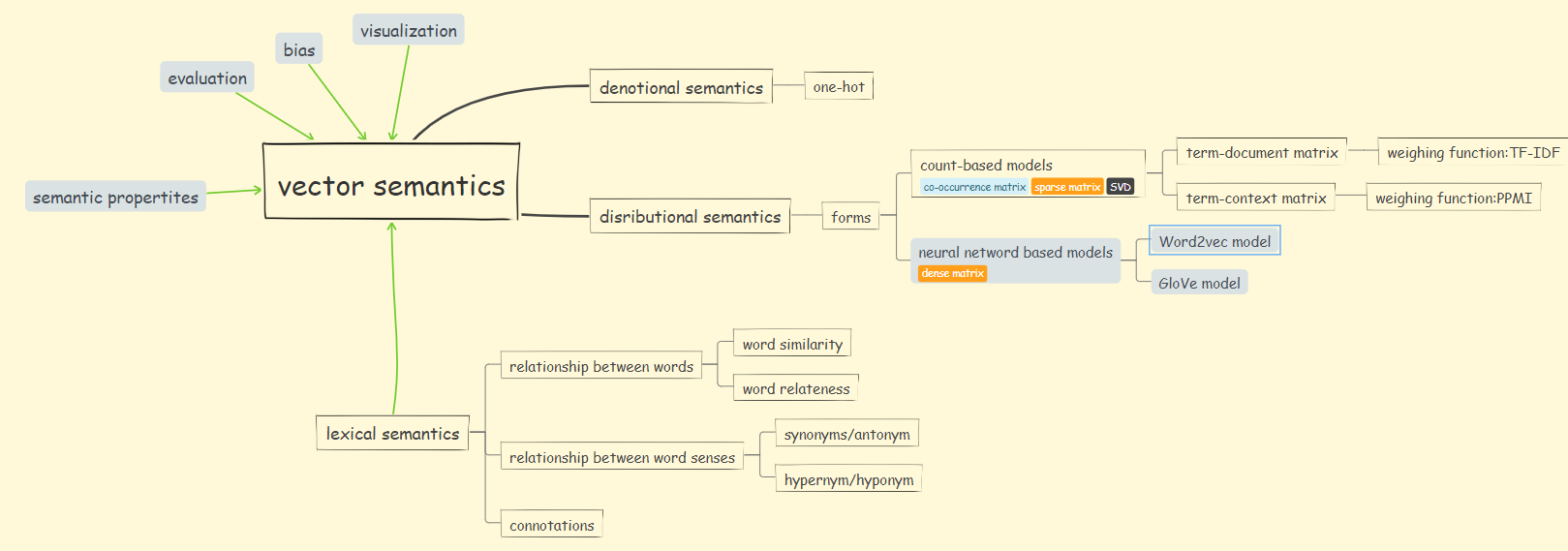### Lexical Semantics

1. Lemmas and Senses
mouse: 词条(leamma or citation form)
mice: 词条的特殊形式如单复数、过去式等称为单词形式(wordforms)
rodent/cursor control device: 一个词条可有多个词义(word sense)
2. Relationships between senses
同义词(synonyms): 我们都知道含义相同或基本相同为同义词。语言学中更正式的定义是两个词在任意句子中可相互替代且不改变句子的真值，则这两个词具有相同的命题含义(propositional meaning)。但事实上没有含义绝对相同的两个词。语义学中的一个基本原则叫对比原则(principle of contrast):语言形式的差异至少与含义上的差异有关。比如$H_2$O虽然和water都是“水”的意思，但前者更多用于科学资料中，用在某份远行指南中就不合适了。因此我们常说的同义词只是去通常描述词义大致相同的情况。
反义词(antonym)
上义词(hypernym)
下义词(hyponym):和上义词一起，其实描述的是一种IS-A的词等级关系。
3. Relationships between words
♦ Word Similarity
尽管词没有很多同义词，但是大多数词都有很多相似词(similar words)。比如cat和dog虽然不是同义词但是却是相似词。从同义词到相似性，从讨论词义之间的关系(如同义词)到讨论词与词之间的关系(如相似性)是很有帮助的。处理单词时避免了对词义的特定表示，这将简化我们的任务。从词相似性到短语相似性到句子相似性，这对处理大型的语义任务是很有帮助的。
词相似性描述共有某些特征(share some features)的词。
比如在SimLex-999数据集中(Hill,2015)给出了0-10的范围值来判定词相似度。
♦ Word Relatedness
词关联性描述共同出现在同一语境中或相同语义场(co-participate in a shared environment or the semantic field)中
一个semantic field是一组包含特定语义域的词，它们之间具有结构化关系。比如hospital的语义场包含的词有surgeon、nurse、scalpel等。
4. Connotation
即情感含义(affective meaning)。
早期关于情感意义的研究(Osgood,1957)发现，词汇在情感意义的三个重要维度上是不同的——valence、arousal和dominance，定义如下:
valence: the pleasantness of the stimulus
arousal: the intensity of emotion provoked by the stimulus
dominance: the degree of control exerted by the stimulus
另外，Osgood还提出一个很有进展性的想法————可以用一串数字表示词的含义，并且可以表示为空间中的一个点。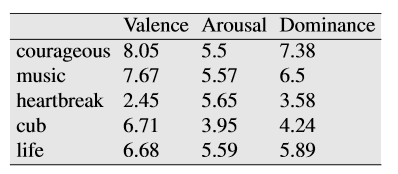### Words and Vectors

1. document vectors
其实就是以字典中的词作为行，语料库中的文档作为列构建的term-document co-occurrence matrix。最初用于文档信息检索中的查找相似文档的任务。这时一个文档用一个列向量去表示。可以想见这种matrix是稀疏且庞大的。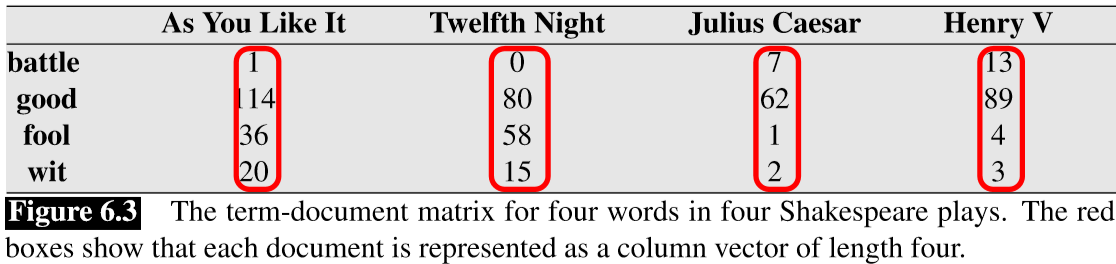2. word vectors
以字典中的词作为行，以语料库中的文档或context word作为列构建的term-context matrix。这时，一个词用一个行向量去表示。同样，这种matrix也是稀疏和耗费存储空间的。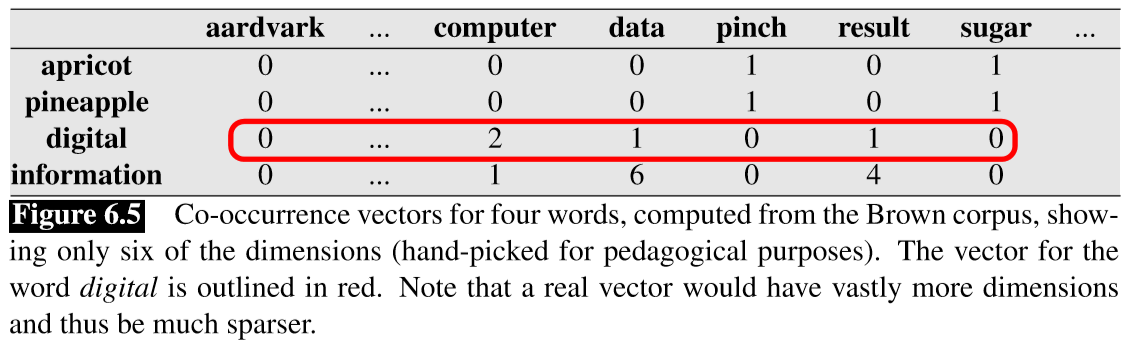3. cosine for measuring similarity
此方法是计算词向量相似度最常用的方法。
向量点积(dot product)/内积(inner product)的计算公式为：
$\vec{v}\cdot\vec{w}=\sum_{i=1}^Nv_iw_i=v_1w_1+v_2w_2+…+v_Nw_N=|\vec{v}||\vec{w}|cos\theta$
因此，计算两个向量cosine相似性的公式为：
$cosine(\vec{v},\vec{w})=\frac{\vec{v}\cdot\vec{w}}{|\vec{v}||\vec{w}|}=\frac{\sum_{i=1}^Nv_iw_i}{\sqrt{\sum_{i=1}^Nv_i^2}\sqrt{\sum_{i=1}^Nw_i^2}}$
也就是说，两个词向量的相似度可以用其cosine值来表示，在0~1之间。值越大,两向量距离越小，说明两个词向量越相似。举例如下：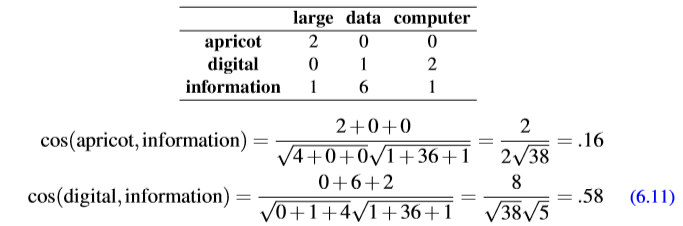### TF-IDF：Weighing terms in the vector

TF-IDF是一种用于信息检索与文本挖掘的常用加权技术，并公认为是信息检索中最重要的发明。tf-idf是一种统计方法，用以评估一字词对于一个文件集或一个语料库中的其中一份文件的重要程度。字词的重要性随着它在文件中出现的次数成正比增加，但同时会随着它在语料库中出现的频率成反比下降。

1. 词频term frequency(Luhn,1957)：the frequency of the word in the doc.其中，$count(w_t,d)$为词$w_t$在文档d中出现的次数，$count(w,d)$为文档d的总词数。
2. 逆文档频率inverse document frequency(Sparck Jones,1972)其中，D为文档总数，$D_{w_t}$为包含词$w_t$的文档数。
这里解释下为什么用对数：若是简单的取逆处理，通用词的权重会非常小，稀缺词权重过大，因此采用取对数。
3. 计算tf-idf

♦ 不足
TF-IDF由于其简单结构造成对权值调整不是很好，精度并不是很高；此外在TF-IDF中并没有体现出单词的位置信息，把文档中的词都看作独立的个体进行处理，忽略了词的意义、词和词之间的关联关系。比如对于Web文档而言，权重的计算方法应该体现出HTML的结构特征。特征词在不同的标记符中对文章内容的反映程度不同，其权重的计算方法也应不同。因此应该对于处于网页不同位置的特征词分别赋予不同的系数，然后乘以特征词的词频，以提高文本表示的效果。(引自维基百科https://zh.wikipedia.org/wiki/Tf-idf)
♦ 注意

### Pointwise Mutual Information(PMI) or Positive PMI(PPMI)

1. PMI(Fano,1961)
逐点互信息是基于两个随机变量X和Y的互信息(mutual information)，即当时，Fano把我们现在称为逐点互信息的称为互信息，也就是$I(x,y)=log_2\frac{P(x,y)}{P(x)P(y)}$。把我们称为互信息的称为互信息的期望，也就是上面列出的式子$I(X,Y)$。
于是中心词(target word) $w$和上下文词(context word) $c$之间的逐点互信息(Church and Hanks 1989)定义为：$PMI(w,c)=log_2\frac{P(w,c)}{P(w)P(c)}$
分子告诉我们观察到的两个单词同时出现的频率(假设我们使用MLE计算概率)。分母告诉我们，如果这两个单词各自独立出现，我们期望它们同时出现的频率;两个独立事件同时发生的概率是两个事件概率的乘积。因此，这个比例给了我们一个估计值，即这两个词同时出现的概率比我们预期的要高多少。PMI是一个有用的工具来找到关联密切的词。
2. PPMI(Church and Hanks 1989)
PMI的取值是($+\infty,-\infty$)。首先，PMI取负值表示的是两个词同时出现的频率比我们期望它们同时出现的频率少多少。这个结果除非是语料库很大否则是很不可靠的。其次，PMI取负值说明的是两个词的不相关程度。所以，最常用的是Postive PMI(PPMI)，也就是用0替代所有PMI的负值：假如有$W$行(words)和$C$列(contexts)的共现矩阵$F$，$f_{ij}$为中心词$w_i$和上下文词$c_j$同时出现的次数。转化为PPMI矩阵，其中$ppmi_{ij}$给出上下文词$c_j$下中心词$w_i$的PPMI值为:因此，$PPMI_{ij}=max(log_2\frac{p_{ij}}{p_{i*}p_{*j}},0)$
3. Example
基于上文的term-context matrix，我们计算PPMI(w=information,c=data)：
$P(w=information,c=data)=\frac{6}{19}=.316$
$P(w=information)=\frac{11}{19}=.579$
$P(c=data)=\frac{7}{19}=.368$
$ppmi(w=information,c=data)=log_2(.316/(.368*.579))=.568$
以此类推，可得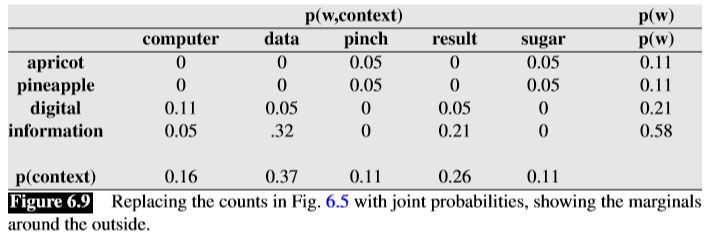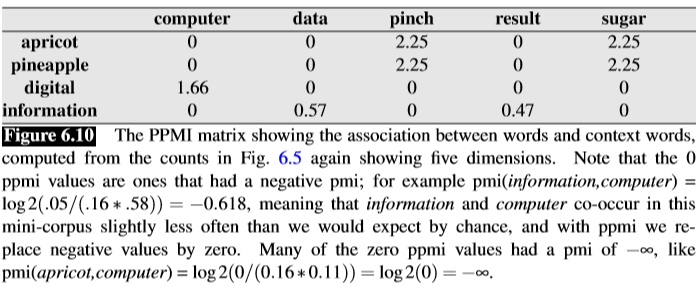4. Extensions：
PMI存在着偏斜不频繁事件的问题;非常罕见的词汇往往具有非常高的PMI值。
♦ 减少这种倾向于低频率的事件的一个方法是稍微改变$P(c)$的计算,使用不同的函数$P(c)$,提出了上下文$P_{\alpha}(c)$:其中，Levy(2015)发现α= 0.75时，在很多任务中表现有明显提高。因为α= 0.75增加概率分配到罕见的context上,因此降低了其PMI ($P_{\alpha}(c)$> P (c)，当c是rare context)。
♦ 另一个可能的解决方法是拉普拉斯平滑(Laplace smoothing):在计算PMI之前，在每个计数中添加一个小的常数k(通常为0.1-3)，收缩所有的非零值。k越大，非零值的折扣就越大。